## Semiperimeter

The semiperimeter on a figure is defined as(1)

whereis the Perimeter. The semiperimeter of Polygons appears in unexpected ways in the computation of their Areas. The most notable cases are in the Altitude, Exradius, and Inradius of a Triangle, the Soddy Circles, Heron's Formula for the Area of a Triangle in terms of the legs,, and(2)

and Brahmagupta's Formula for the Area of a Quadrilateral(3)

The semiperimeter also appears in the beautiful L'Huilier's Theorem about Spherical Triangles.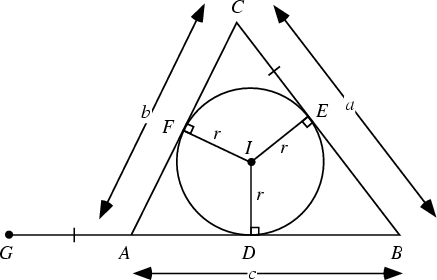For a Triangle, the following identities hold,(4)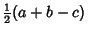(5)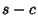(6)

Now consider the above figure. Letbe the Incenter of the Triangle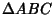, with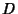,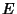, andthe tangent points of the Incircle. Extend the line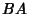with. Note that the pairs of triangles,,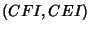are congruent. Then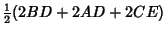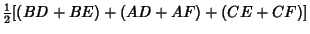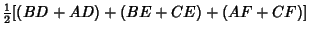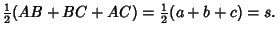(7)

Furthermore,(8)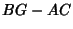(9)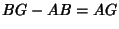(10)

(Dunham 1990). These equations are some of the building blocks of Heron'sderivation of Heron's Formula.

References

Dunham, W. Heron's Formula for Triangular Area.'' Ch. 5 in Journey Through Genius: The Great Theorems of Mathematics. New York: Wiley, pp. 113-132, 1990.